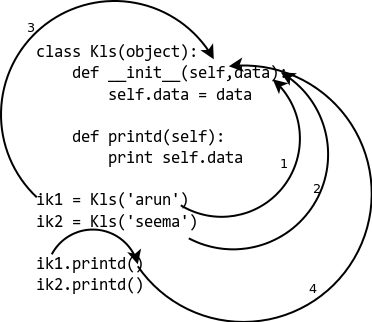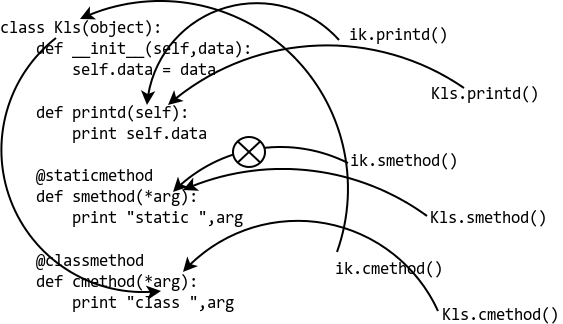"
Last Updated: Wednesday 29th December 2021

## Class vs Static Methods in Python

In this article I'll try to explain what are `staticmethod` and `classmethod`, and what the difference is between them. `staticmethod` and `classmethod` both use decorators for defining a method as a `staticmethod` or `classmethod`. Please take a look at the article Python Decorators Overview for a basic understanding of how decorators works in Python.

## Simple, Static and Class Methods

The most used methods in classes are instance methods, i.e instance is passed as the first argument to the method.

For example, a basic instance method would be as follows:

[python]
class Kls(object):
def __init__(self, data):
self.data = data

def printd(self):
print(self.data)

ik1 = Kls('arun')
ik2 = Kls('seema')

ik1.printd()
ik2.printd()
[/python]

This gives us the following output:

[shell]
arun
seema
[/shell]After looking at the code sample and diagram:

• In 1 and 2, the arguments are passed to the method.
• On 3, the `self` argument refers to the instance.
• At 4, we do not need to provide the instance to the method, as it is handled by the interpretor itself.

Now what if the method we want to write interacts with classes only and not instances? We can code a simple function out of the class to do so but that will spread the code related to class, to out of the class. This can cause a future code maintenance problem, as follows:

[python]
def get_no_of_instances(cls_obj):
return cls_obj.no_inst

class Kls(object):
no_inst = 0

def __init__(self):
Kls.no_inst = Kls.no_inst + 1

ik1 = Kls()
ik2 = Kls()

print(get_no_of_instances(Kls))
[/python]

Gives us the following output:

[shell]
2
[/shell]

## The Python @classmethod

What we want to do now is create a function in a class, which gets the class object to work on instead of the instance. If we want to get the no of instances, all we have to do is something like below:

[python]
def iget_no_of_instance(ins_obj):
return ins_obj.__class__.no_inst

class Kls(object):
no_inst = 0

def __init__(self):
Kls.no_inst = Kls.no_inst + 1

ik1 = Kls()
ik2 = Kls()
print iget_no_of_instance(ik1)

[/python]

[shell]
2
[/shell]

Using features introduced after Python 2.2, we can create a method in a class, using `@classmethod`.

[python]
class Kls(object):
no_inst = 0

def __init__(self):
Kls.no_inst = Kls.no_inst + 1

@classmethod
def get_no_of_instance(cls_obj):
return cls_obj.no_inst

ik1 = Kls()
ik2 = Kls()

print ik1.get_no_of_instance()
print Kls.get_no_of_instance()
[/python]

We get the following output:

[shell]
2
2
[/shell]

The benefit of this is: whether we call the method from the instance or the class, it passes the class as first argument.

## The Python @staticmethod

Often there is some functionality that relates to the class, but does not need the class or any instance(s) to do some work. Perhaps something like setting environmental variables, changing an attribute in another class, etc. In these situation we can also use a function, however doing so also spreads the interrelated code which can cause maintenance issues later.

This is a sample case:

[python]
IND = 'ON'

def checkind():
return (IND == 'ON')

class Kls(object):
def __init__(self,data):
self.data = data

def do_reset(self):
if checkind():
print('Reset done for:', self.data)

def set_db(self):
if checkind():
self.db = 'new db connection'

ik1 = Kls(12)
ik1.do_reset()
ik1.set_db()
[/python]

Which gives us the following output:

[shell]
Reset done for: 12
[/shell]

Here if we use a `@staticmethod`, we can place all code in the relevant place.

[python]
IND = 'ON'

class Kls(object):
def __init__(self, data):
self.data = data

@staticmethod
def checkind():
return (IND == 'ON')

def do_reset(self):
if self.checkind():
print('Reset done for:', self.data)

def set_db(self):
if self.checkind():
self.db = 'New db connection'
print('DB connection made for: ', self.data)

ik1 = Kls(12)
ik1.do_reset()
ik1.set_db()
[/python]

Which gives us the following output:

[shell]
Reset done for: 12
[/shell]

Here is a more comprehensive code example, with a diagram to show you

## How @staticmethod and @classmethod are different.

[python]
class Kls(object):
def __init__(self, data):
self.data = data

def printd(self):
print(self.data)

@staticmethod
def smethod(*arg):
print('Static:', arg)

@classmethod
def cmethod(*arg):
print('Class:', arg)
[/python]
[python]
>>> ik = Kls(23)
>>> ik.printd()
23
>>> ik.smethod()
Static: ()
>>> ik.cmethod()
Class: (<class '__main__.Kls'>,)
>>> Kls.printd()
TypeError: unbound method printd() must be called with Kls instance as first argument (got nothing instead)
>>> Kls.smethod()
Static: ()
>>> Kls.cmethod()
Class: (<class '__main__.Kls'>,)
[/python]

Here's a diagram to explain what's going on: